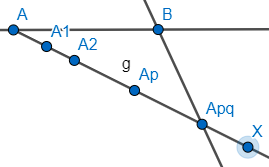Courses
Courses for Kids
Free study material
Free LIVE classes
More# To divide a line segment AB in the ratio p: q, draw a ray AX, so that $\angle$BAX is an acute angle and then mark points on ray AX at equal distances such that the minimum number of these points is:(a) Greater of p and q(b) p+q(c) p+q-1(d) pq

Last updated date: 19th Mar 2023
Total views: 303.9k
Views today: 4.83kVerified
303.9k+ views
Hint: To solve this problem, we need to be aware about the basic construction concepts related to dividing a line into equal parts. Thus, in case of line segment AB, to get the ratio of p: q, we divide the line segment into a certain number of points such that we are able to divide AB into the desired ratio p: q.

To solve this problem, we start with drawing a line segment AB. We then draw ray AX such that it should make an acute angle with line segment AB and then mark p+q points at equal units on the ray AX. To explain, we draw and mark these p+q points in the following manner with the help of arcs as shown (in the figure there are 5 points, in our case, there will be p+q points). In the below figure, ${{A}_{pq}}$represents ${{A}_{\begin{smallmatrix} p+q \\ \end{smallmatrix}}}$.Thus, in our case, the points would be ${{A}_{1}},{{A}_{2}},....,{{A}_{p+q}}$. We will then draw a line segment from point ${{A}_{p+q}}$ to B. Our next step would be to draw a parallel line segment to line segment XB from the point ${{A}_{p}}$. Thus, we get,
Thus, line segment ${{A}_{p}}C$ divides AB in ratio p:q.